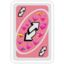MathematicsOpenStudy (anonymous):

Differentiate using product rule:OpenStudy (anonymous):

Here is the question: $h(x)=(x^2+5x+7).(x^3+2x-4)$OpenStudy (anonymous):

right? $(2x+5)(3x^2+2)$OpenStudy (campbell_st):

let $u = x^2 + 5x + 7 ===> \frac{du}{dx} = 2x + 5$ $v = x^3 + 3x - 4 ===> \frac{dv}{dx} = 3x^2 + 3$ then $\frac{dy}{dx} = u \times \frac{dv}{dx} + v \times \frac{ du}{dx}$OpenStudy (campbell_st):

just substitute and simplifyOpenStudy (anonymous):

(f(x).g(x))' = f(x).g'(x) + g(x).f'(x)OpenStudy (anonymous):

yes let me do it. $(x^2+5x+7) (3x^2+2) + (2x+5)(x^3+2x-4)$OpenStudy (anonymous):

is that right?OpenStudy (anonymous):

yup. looks right to meOpenStudy (anonymous):

Now, I am confuse with the next part. I cannot simplify. :(OpenStudy (campbell_st):

normally you would be expected to simplify.... not sure on your question requirementsOpenStudy (anonymous):

yes I have to simplify it after applying product rule.OpenStudy (campbell_st):

a simple method is to draw up a grid |dw:1338113768920:dw|

Latest QuestionsTyrion: Define love
8 minutes ago 7 Replies 2 Medalsogzec: Aristotle influenced medieval times. Describe the ideas inspired or borrowed from Aristotle.
34 minutes ago 0 Replies 0 Medalsdestiny14: Sarabeth used a box-and-whisker plot to summarize her race times in minutes. She didn't include her latest race time of 28 minutes.
41 minutes ago 0 Replies 0 MedalsBrookiecookie: Just need help with the last three Prompt: Now that you have finished reading the works in this unit, consider the ideals, values, and themes that early Ame
48 minutes ago 0 Replies 0 Medalskylieg123: An ice sculpture is melting from the heat. Assuming its height changes by negativ
1 hour ago 1 Reply 0 MedalsYuri: Oni was timing her 100 meter sprints. Her first five sprints were 12.568 seconds, 11.
1 hour ago 0 Replies 0 Medalsjc17: If you like to make logos and would like to make a logo for question cove this is what I'm using.
1 hour ago 9 Replies 1 Medalkayleigh344: Which expression can be used find 40% of 130?
1 hour ago 0 Replies 0 Medalsjc17: witch question cove logo do yall like?...
1 hour ago 10 Replies 1 Medalyesimfuny: How do you help yourself focused?
2 hours ago 9 Replies 1 Medal